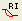RocFall3 | Your new tool for 3D assessment of slopes at risk for rockfalls. Get started with RocFall3 today!

# Critical Probabilistic Surface

If the Probabilistic Analysis Type = Overall Slope, then the Critical Probabilistic slip surface can be displayed with the Show Critical Probabilistic Surfaceoption in the toolbar or the Statistics menu. This is the slip surface, of all slip surfaces analyzed, which has the Minimum Reliability Index. By definition, this slip surface also has the Maximum Probability of Failure.

The Critical Probabilistic Surface WILL NOT NECESSARILY BE THE SAME AS THE CRITICAL DETERMINISTIC SLIP SURFACE (i.e. the slip surface with the lowest safety factor when all input parameters are equal to their mean value). In general, the Critical Probabilistic Surface and the Critical Deterministic Surface (i.e. the deterministic Global Minimum slip surface), can be different surfaces.

Also, the RI of the Critical Probabilistic Surface will NOT be equal to the RI which is obtained using the aggregate safety factor data of all of the different Global Minimum slip surfaces which are located by the Overall Slope analysis.

• The RI of the Critical Probabilistic Surface will always be GREATER THAN the RI for the entire analysis (i.e. the RI displayed in the Legend).
• The PF of the Critical Probabilistic Surface will always be LESS THAN the PF for the entire analysis (i.e. the PF displayed in the Legend).

The Critical Probabilistic Surface, therefore provides a less conservative measure of the probabilistic slope stability, compared to the results reported using ALL Global Minimum surfaces located by the Overall Slope analysis.

The Critical Probabilistic Surface will NOT be calculated, for the following search options.

## Auto Refine Search

The Critical Probabilistic Surface will NOT be available if you have used the Auto Refine Search method (for circular slip surfaces). This is because the Auto Refine Search method generates DIFFERENT slip surfaces, each time the analysis is run, using different input data. Therefore, the Critical Probabilistic Surface cannot be calculated, because the slip surfaces change with each run of the Auto Refine Search method. (In order to calculate the Critical Probabilistic slip surface, it is necessary that the slip surfaces which are generated by the search, ARE THE SAME SURFACES, for each iteration of the search. This is the case for all search methods in Slide2, except the Auto Refine Search method).

## Optimized Surfaces

If you have used the Optimization search option (selected in the Surface Options dialog in the Slide2 Model program), then the Critical Probabilistic Surface will NOT INCLUDE the optimized surfaces. Again, this is because the optimized surface changes each time the analysis is run, using different input data. However, if you have used the Optimization option, the Critical Probabilistic Surface WILL BE CALCULATED using all of the original surfaces generated by your initial search method (e.g. Block Search or Path Search).